Question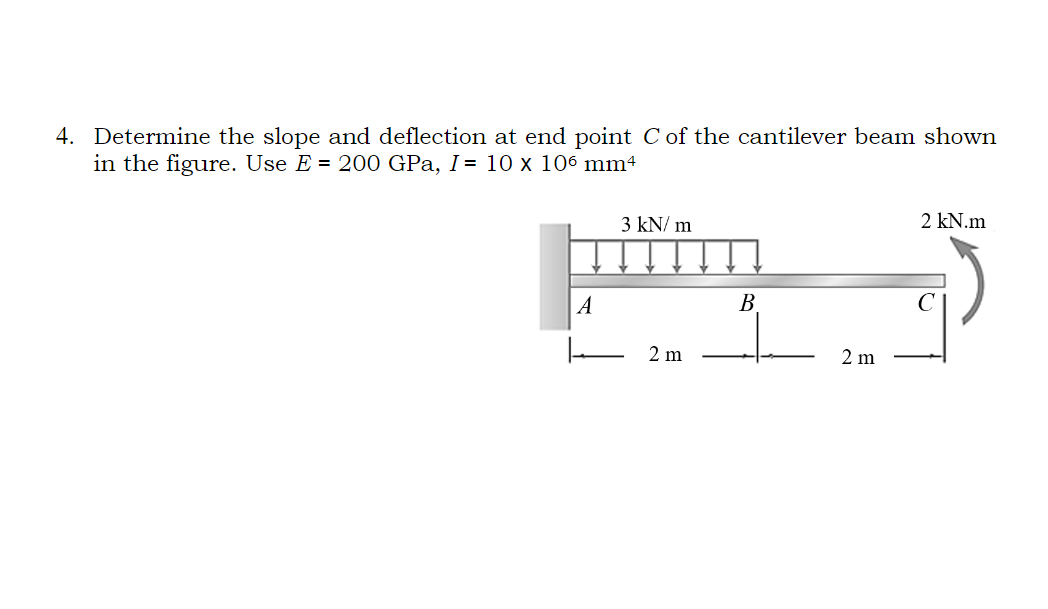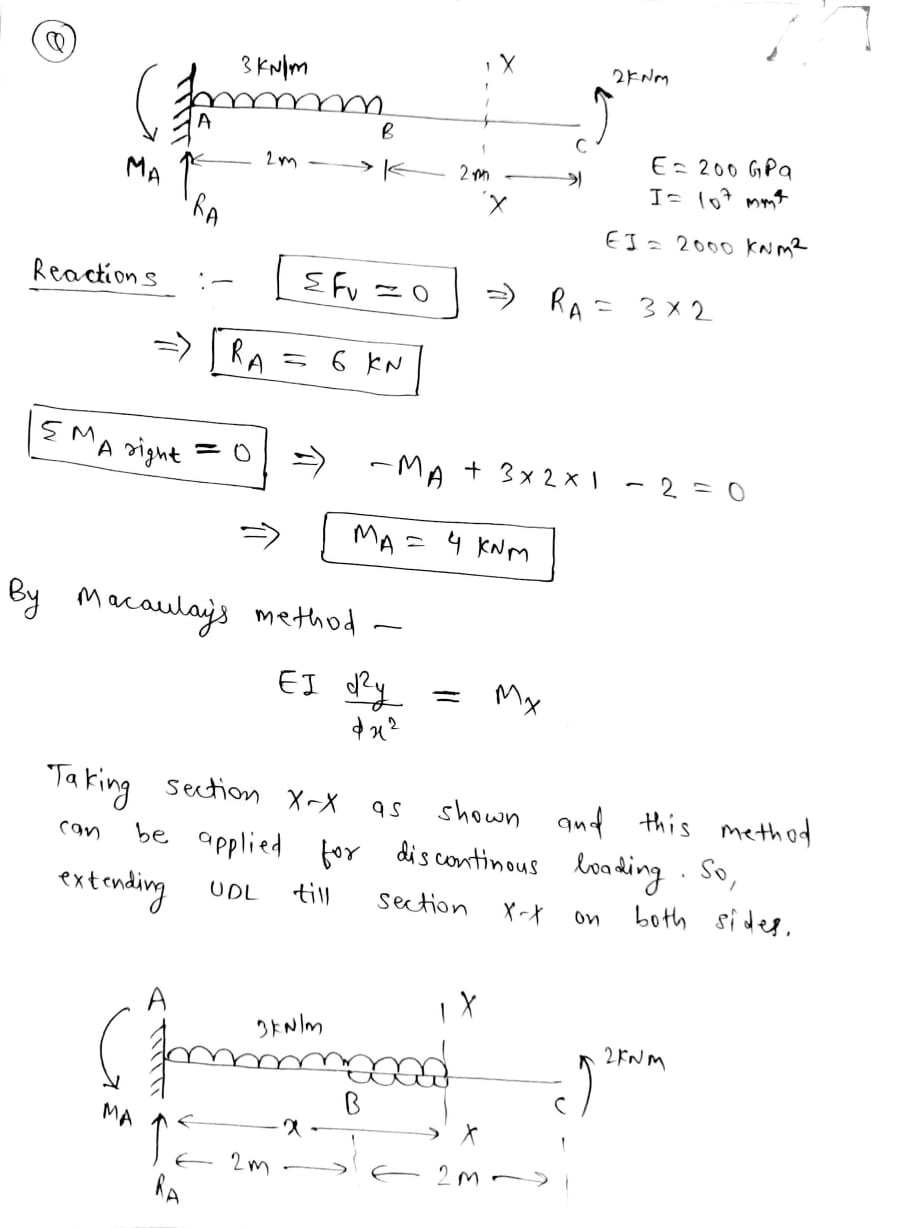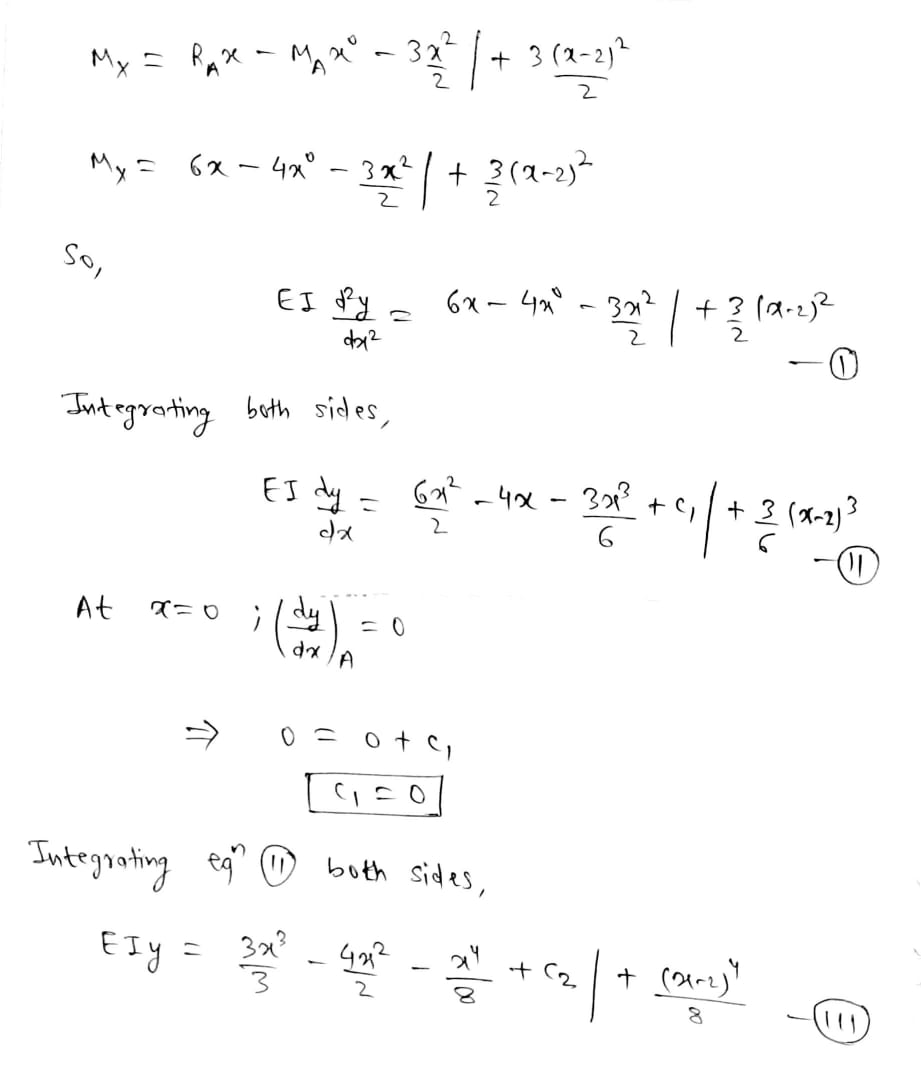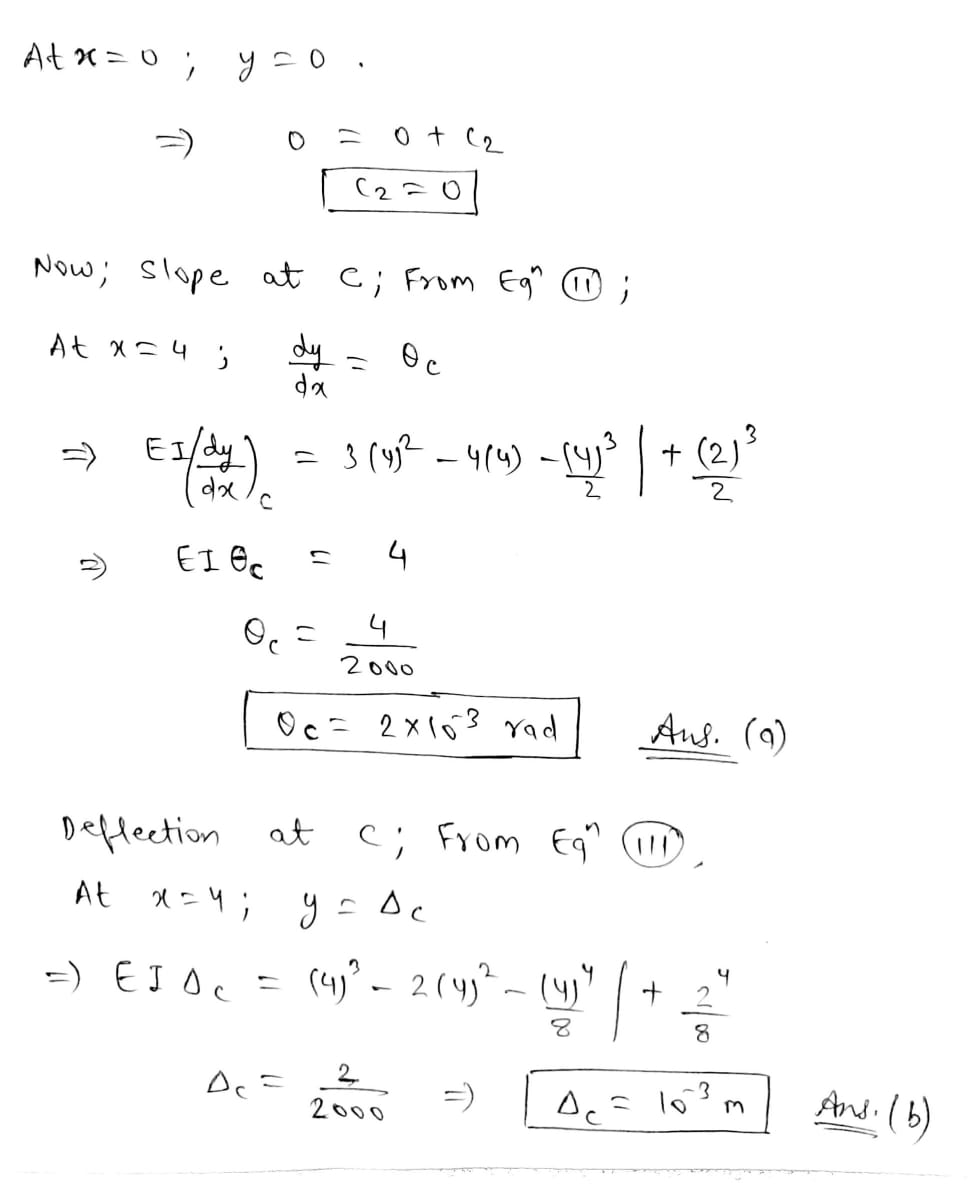Doubts and Queries are welcomed in the comment box! Thank you !

#### Earn Coins

Coins can be redeemed for fabulous gifts.

Similar Homework Help Questions
• ### For the cantilever beam and loading shown, determine the slope and deflection at point B. Use...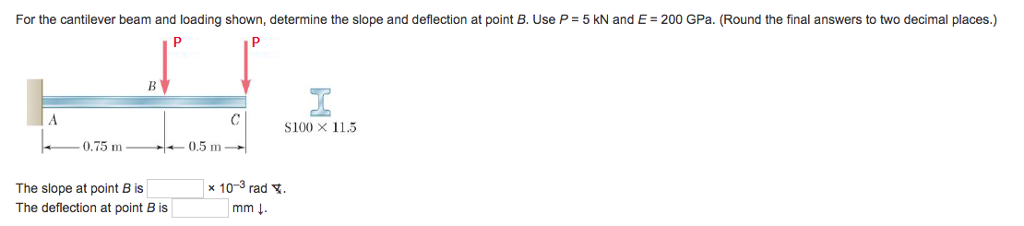For the cantilever beam and loading shown, determine the slope and deflection at point B. Use P 5 kN and E 200 GPa. (Round the final answers to two decimal places.) S100 X 11.5 0.75 m 0.5 m The slope at point B is The deflection at point B is x 10-3 rad. mm ↓

• ### Check my work For the cantilever beam and loading shown, determine the slope and deflection at...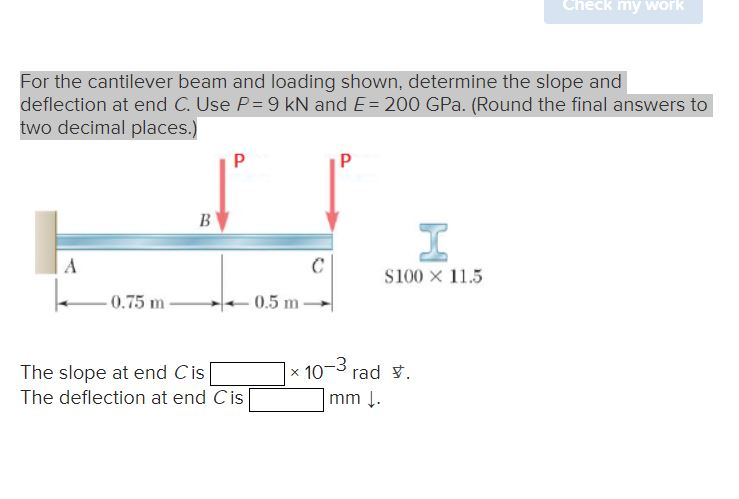Check my work For the cantilever beam and loading shown, determine the slope and deflection at end C. Use P = 9 kN and E= 200 GPa. (Round the final answers to two decimal places.) P Р B I A \$100 X 11.5 -0.75 m 0.5 m The slope at end Cis The deflection at end Cis x 10m rad . -3 mm.

• ### Problem 3: For the beam shown find the slope and deflection at point B and C...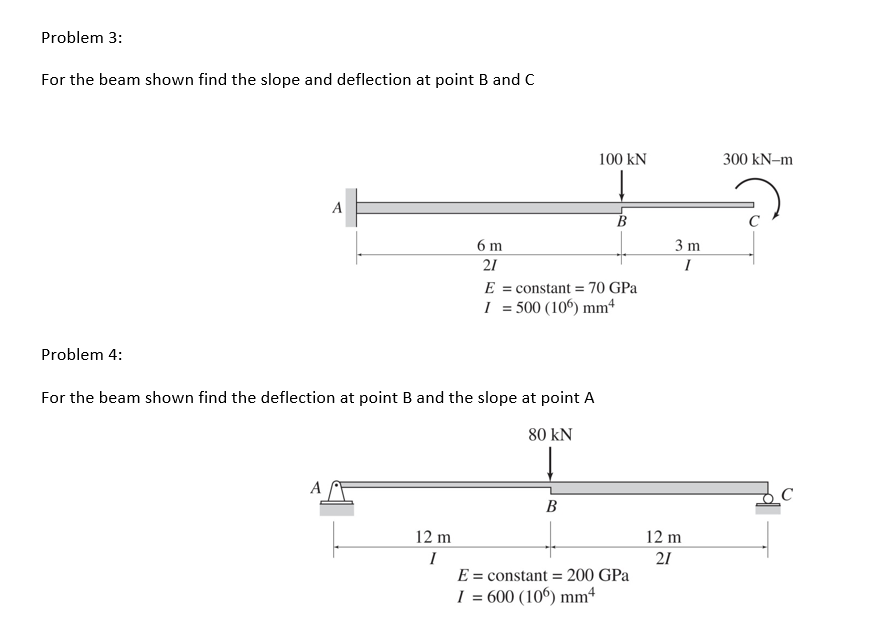Problem 3: For the beam shown find the slope and deflection at point B and C 100 KN 300 kN-m 6 m E = constant = 70 GPa 1 = 500 (106) mm Problem 4: For the beam shown find the deflection at point B and the slope at point A 80 KN 12 m 12 m E = constant = 200 GPa I = 600 (106) mm

• ### For the cantilever beam and loading shown, determine the slope and deflection at end C. Use...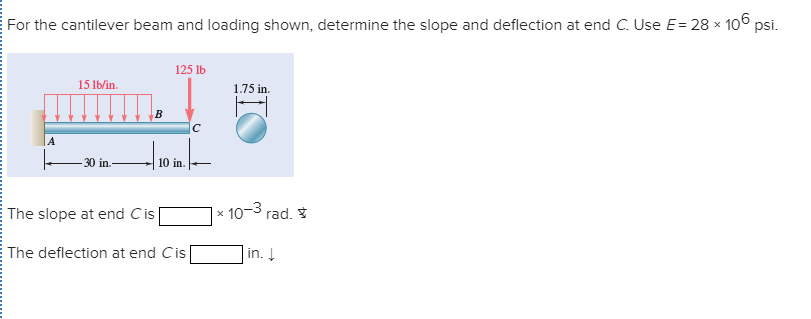For the cantilever beam and loading shown, determine the slope and deflection at end C. Use E= 28 × 106 psi. 125 lb 15 lb/in. 1.75 in. |A 30 in. 10 in. x 10-3 The slope at end Cis rad. * in. Į The deflection at end Cis

• ### For the cantilever beam and loading shown, determine the slope and deflection at point B. Use...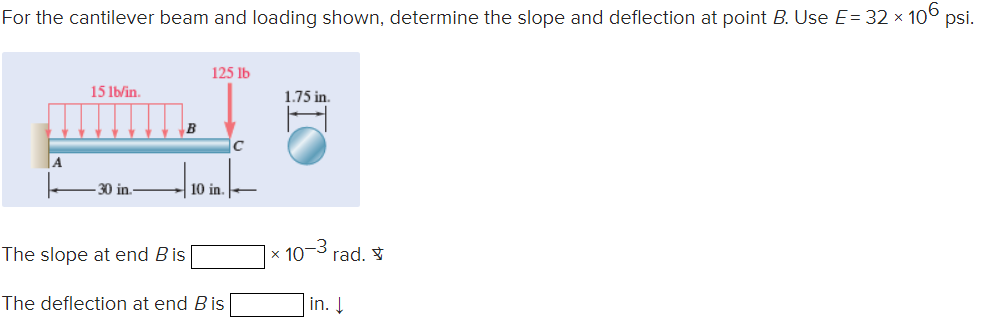For the cantilever beam and loading shown, determine the slope and deflection at point B. Use E = 32 x 106 psi. 125 lb 15 lb/in 1.75 in B 30 in 10 in. The slope at end Bis x 107 rad. The deflection at end B is in.

• ### For the cantilever steel beam shown below, find the deflection at point B. Q5 Assume that:...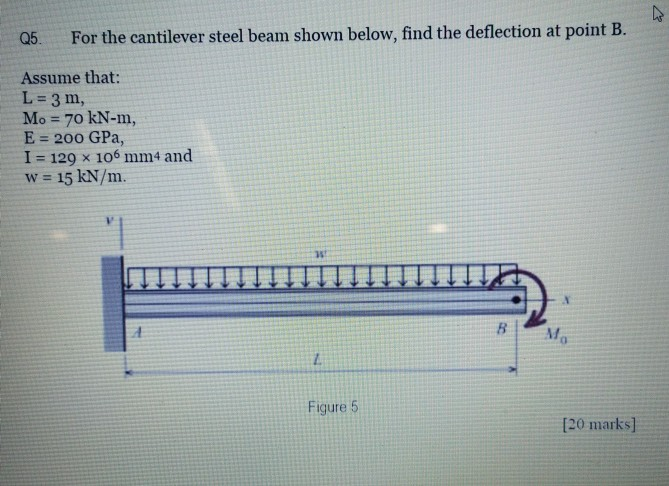For the cantilever steel beam shown below, find the deflection at point B. Q5 Assume that: L 3 m, Mo 70 kN-m, E 200 GPa, I 129 x 106 mm4 and w= 15 kN/m. Mo Figure 5 [20 marks]

• ### 2. For the beam and loading shown, determine the slope and deflection at point B. Where:...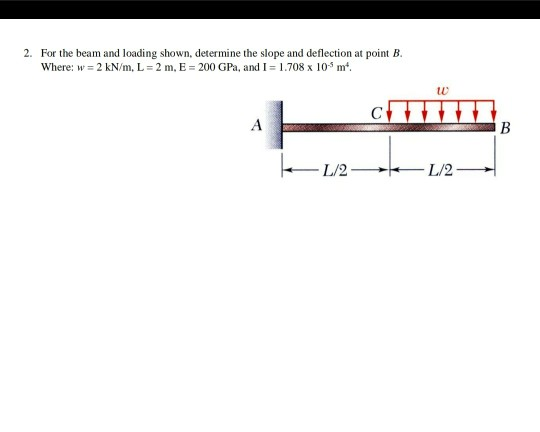2. For the beam and loading shown, determine the slope and deflection at point B. Where: w = 2 kN/m, L = 2 m, E = 200 GPa, and I = 1.708 x 10 m. B 1/2- 1/2

• ### The simply supported beam consists of a w530 x 66 structural steel wide-flange shape [E-200 GPa; I -351 x 106 mm]. Determine (a) the beam deflection at point C. (b) the beam deflection at point E. As...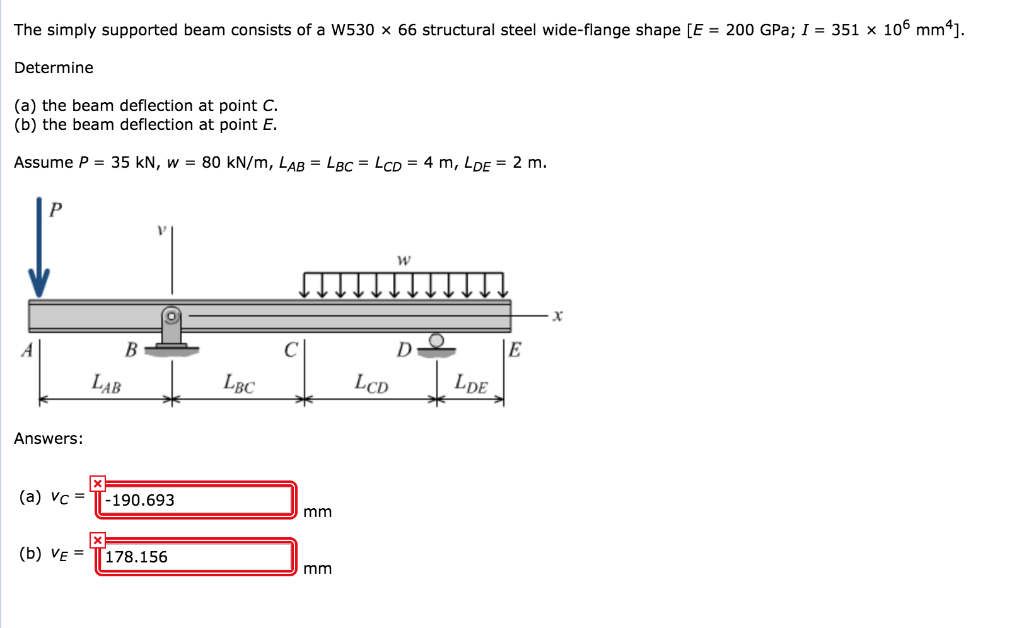The simply supported beam consists of a w530 x 66 structural steel wide-flange shape [E-200 GPa; I -351 x 106 mm]. Determine (a) the beam deflection at point C. (b) the beam deflection at point E. Assume P = 35 kN, w = 80 kN/m, LAB = LBC = LCD = 4 m, LDE = 2 m LAB BC Answers: (a) vc=T-190.693 (b) VE178.156 The simply supported beam consists of a w530 x 66 structural steel wide-flange shape [E-200 GPa;...

• ### A cantilever beam of a channel section is loaded at its half-length, as shown in Figure Q2. The Young's modulus of the material is 200 GPa. Determine the deflection at the free end. [12.5 marks]...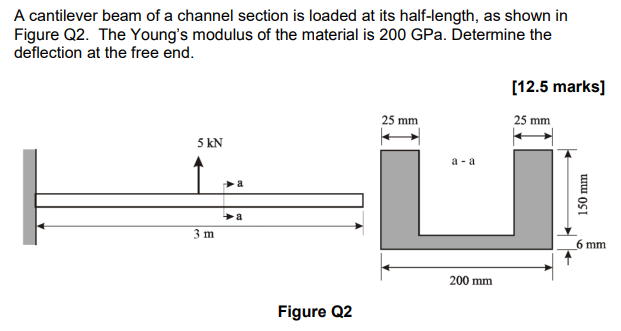A cantilever beam of a channel section is loaded at its half-length, as shown in Figure Q2. The Young's modulus of the material is 200 GPa. Determine the deflection at the free end. [12.5 marks] 25 mm 25 mm 5 kN a -a 少a 6 mm 200 mm Figure Q2 A cantilever beam of a channel section is loaded at its half-length, as shown in Figure Q2. The Young's modulus of the material is 200 GPa. Determine the deflection at...